#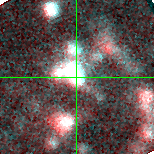M31-003944.71 (J003944.70+402056.1)

## Target Notes### Classification: HII

Resolved from four LGGS stars combining to comparable brightness or brighter 2.2 arcsec to the NE. Marked B or X when not clearly resolved.

## Plots of Brightness and Color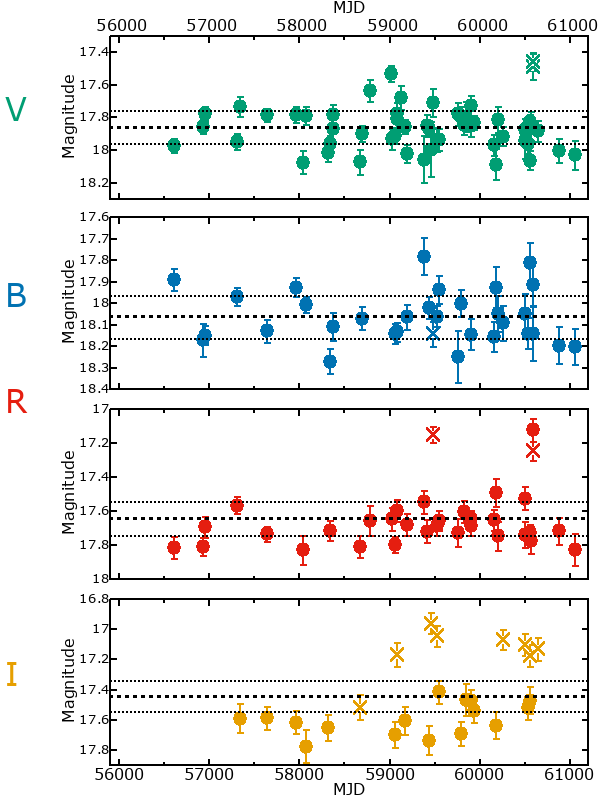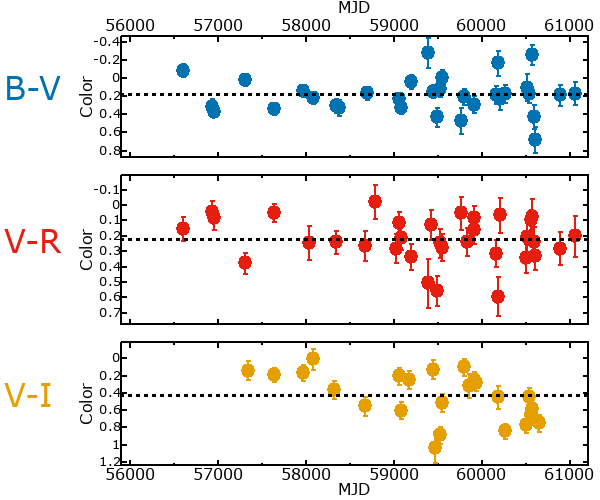## Variability

From the data available in this survey, it is not possible to tell with certainty if this star is variable.

### Correlation factors between bands

If the brightness variation is true variability then there should be a correlation between brightness changes recorded in different filter bands. The following table are the R-squared linear correlation factors calculated for the pairs of bands. The number in parentheses next to the R-squared value is the number of elements used to calculate the correlation factor. The number in square brackets next to the band is the range of magnitudes measured.

V [0.75 mag]B [0.50 mag]R [0.33 mag]
B [0.75 mag]0.11 (15)
R [0.33 mag]0.05 (15)0.30 (11)
I [0.80 mag]0.03 (9)0.09 (4)0.33 (3)

### Magnitude-Magnitude Plots

The plots below are provided to check the values in the table above and also to show any non-linear correlations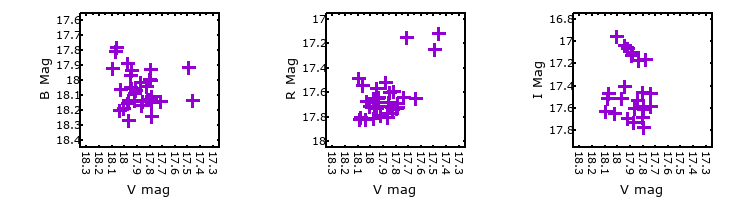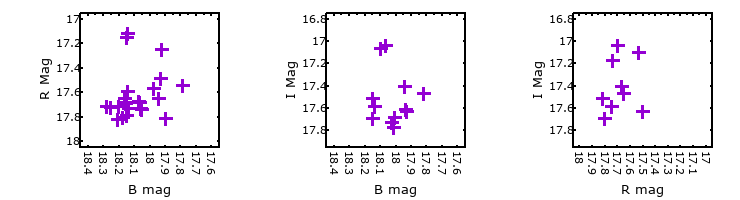### I & R Statistics

Welch & Stetson (1993, AJ, 105, 1813) present a method for varible star detection in CCD images from automated surveys. Data from two bands are compared and two statistics are calculated: R and I.

The I-statistic has an expectation value of zero for stars that are NOT variable. A larger non-zero I-value indicates a higher likelihood that the star is variable. The R statistic indicates how the color of the star changes as the variable grows brighter with R = 1.0 being no change in color.

R and I values have only been calculated if there are more than four (4) matched measurements of brightness in each band.
V
(I|R)
B
(I|R)
R
(I|R)
B
(I|R)
-0.72 | 0.91
R
(I|R)
1.02 | 1.900.60 | 0.31
I
(I|R)
-0.69 | 0.57--- | --- --- | ----